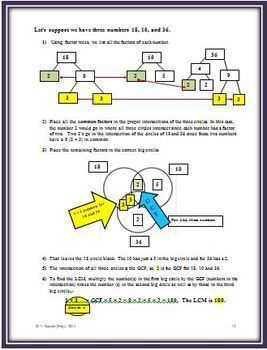# Finding the GCF and LCM for Fractions and Algebra Using a Venn DiagramSubject
Resource Type
Common Core Standards
Product Rating
4.0
1 Rating
File Type

PDF (Acrobat) Document File

1 MB|16 pages
Share
Product Description

This 16 page resource begins with the definitions for the math words factor, greatest common factor and least common multiple, and continues by explaining and illustrating what a factor tree is and how to construct and use a Venn Diagram as a graphic organizer to find the least common multiple.

After finding the GCF, how to find the least common multiple is explained and demonstrated by using the same Venn Diagram. Two pages of step-by-step examples are given, followed by three student practice pages. One of the practice pages is entitled “What’s Missing” and requires the student to read a Venn Diagram to find the two missing numbers, their factors, the LCM and the GCF. A page of 12 blank two circle Venn Diagrams is included.

Also incorporated into this resource is how to use the two circle graphic organizer to factor out the Greatest Common Factor for polynomials or algebraic terms. A detailed example is provided to show how this same process can be used in algebra.

What if there are three numbers? A three circle Venn Diagram would be used. This is fully shown in detail followed by a student practice page. A page of nine blank, three circle Venn Diagrams is added to the resource so that the students can practice.

This resource is an effective and valuable tool when teaching fractions. It is also applicable and helpful in algebra when students are asked to find the LCM or GCF of a set of algebraic terms such as: 25xy, 40xyz. (LCM = 200xyz; GCF = 5xy) or when they must factor out the GCF from a polynomial such as 6x2y3 + 9xy2. An answer key is included.

You might also like these resources:

Using Digital Root and the Divisibility Rules to Help Reduce Fractions

Fractions for the Confused and Bewildered – All Operations

Order of Operations: A New Approach

Simple Math Formulas: Using a Triangular Graphic Organizer

Total Pages
16 pages